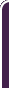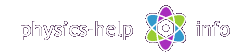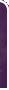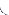homephysics online guidephysics help my info terms of use contactsWhat is Physics? Mechanics Electricity and Magnetism Electric Field Gauss' Law about below subjects Electric Potential Capacity Direct Current Magnetic Field Magnetic Field Laws Magnetic Interactions Electromagnetic Induction Maxwell's Equations Oscillations and Waves Simple Harmonic Motion Damped Harmonic Motion Driven Harmonic Motion Electric Oscillation Alternating Current Wave Motion Elastic Waves Electromagnetic Waves Optics Light Waves Geometrical Optics Interference Polarization Diffraction Fraunhofer Diffraction Dispersion, Absorption, Diffusion Doppler Effect Thermodynamics Ideal Gas Molecular Statistics Transport Phenomena First Law of Thermodynamics Second and Third Laws of Thermodynamics Imperfect Gas Liquids Solids Quantum Physics Thermal Radiation Quantum Properties of Light Wave Properties of Particles Planetary Model of Atom X-Rays Particle in Potential Well Pauli Exclusion Principle Nuclear Physics Solid State Physics Appendices# Noninertial Mechanics

SI units & Physics constants

Noninertial mechanics investigates relative motion of objects inside noninertial reference framesHere (all units see here):

x, y, z is noninertial reference frame with origin at point O

x', y', z' is inertial reference frame with origin at point O'

m is mass of objectis position  vector of noninertial frame with respect to inertial frameis position vector of object with respect to noninertial frameis position vector of object with respect to inertial frameis relative velocity of noninertial frame with respect to inertial frameis relative velocity of object with respect to noninertial frameis relative linear acceleration of object with respect to noninertial frameis linear acceleration of noninertial frameis angular velocity of noninertial frameis angular acceleration of noninertial frame

## General formulas

Noninertial reference frame is a frame of reference which is moving with acceleration

Absolute position vector of object is its position with respect to inertial frame given by vector sumVelocity of noninertial frameRelative velocity of objectAcceleration of noninertial frameRelative acceleration of object## Absolute velocity and acceleration

Velocity of transportation motion is absolute velocity of the point where the object is at the given instant with respect to inertial framewhereis vector product of vectorsandAcceleration of transportation motion is absolute acceleration of the point where the object is at the given instant with respect to inertial frameCoriolis accelerationAbsolute velocity of object with respect to inertial frameAbsolute acceleration of object with respect to inertial frame## Fictitious forces

Total fictious force acting on object in noninertial reference frameNewton's Second Law for motion of object in noninertial reference framewhereis resultant force of all real forces acting on the object from other bodies

## Fictitious force in translating reference frameWhere:

m is mass of objectis acceleration of the systemis net external force applied from other bodiesis fictitious force due to accelerationis relative acceleration of the object with respect to the system

The fictitious force acting on the object due to accelerationEquation for motion of the object inside the system## Fictitious forces in rotating reference frameWhere:is angular velocity of the systemis angular acceleration of the systemis radius of rotation of the objectis relative velocity of the object with respect to the system

The fictitious force acting on the object due to angular acceleration is given by vector productThe centrifugal force due to angular velocityThe Coriolis force due to Coriolis accelerationEquation for motion of object inside the rotating system under the influence of fictious forceswhereis real net external force applied from other bodieshome  |  physics online guide  |  physics help  |  my info  |  terms of use  |  contacts Dr. Nikitin © 2010